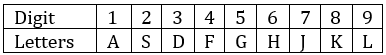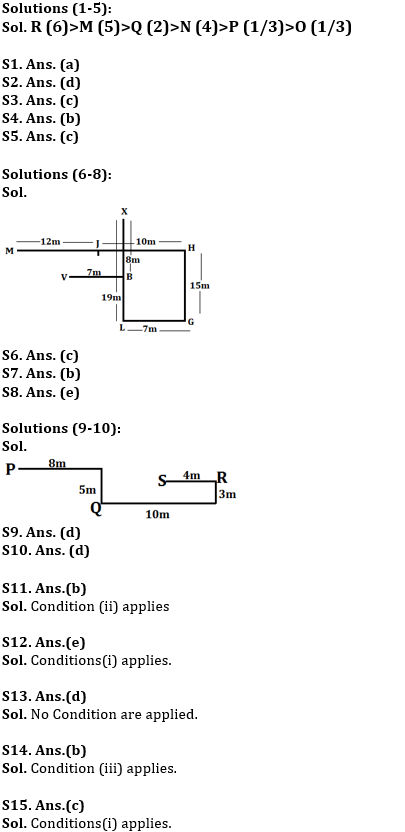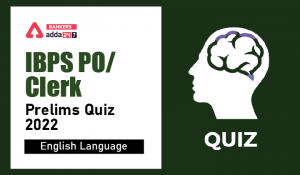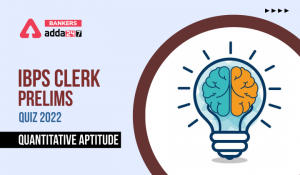Latest Banking jobs   »

# Reasoning Ability Quiz For IBPS Clerk Prelims 2022- 16th July

Directions (1-5): Study the following information and answer the given questions.
There are six boxes having different weight. Each box contains different number of candies- 2, 3, 4, 5, 6 and 1 but not necessarily in the same order.
R contains more candies than M and R’s weight more than O, which contains odd number of candies. Box N contains 4 candies. P weighs more than only O. M weighs more than Q and N, but less than R. Box Q contains 2 candies and Q’s weight is more than N. The difference between the number of candies of box M and N is 1 and Box M contains more than 3 candies.

Reasoning Quiz For IBPS Clerk Prelims 2022 16th July in hindi

Q1. Which of the following box contains highest number of candies?
(a) R
(b) Q
(c) O
(d) M
(e) None of these

Q2. Which of the following box is third heaviest box?
(a) P
(b) O
(c) R
(d) Q
(e) None of these

Q3. What is the difference between number of candies between box R and Q?
(a) 3
(b) 1
(c) 4
(d) 2
(e) None of these

Q4. Which of the following box is heavier than N but lighter than M?
(a) O
(b) Q
(c) R
(d) P
(e) None of these

Q5. How many boxes are lighter than Q?
(a) One
(b) Two
(c) Three
(d) More than Three
(e) None

Directions (6-8): Study the information carefully and answer the questions given below.
Point J is 12m east of point M. Point B is 7m east of Point V. Point J is 10m west of Point H. Point L is 19m south of Point X. Point H is 15m north of Point G which is 7m east of Point L. Point X is 8m north of Point B.

Q6. What is the shortest distance between V and L?
(a) 14m
(b) 13m
(c) √170m
(d) 21m
(e) None of these

Q7. Point V is in which direction with respect to H?
(a) North
(b) South-West
(c) South-East
(d) North-West
(e) West

Q8. What is the shortest distance between Point M and Point V?
(a) 8m
(b) 5m
(c) √65m
(d) 37m
(e) None of these

Directions (9-10): Study the following information carefully to answer the given questions.
A person starts walking from point P in the east direction and walks 8m, then takes a right turn and walks 5m to reached at point Q. From point Q he takes two consecutive left turns and walks 10m and 3m respectively to reach at point R, then finally takes a left turn and walks 4m to reach at point S.

Q9. What is the shortest distance between point S and Q?
(a) 10m
(b) √21m
(c) 16m
(d) √45m
(e) None of these

Q10. What is the direction of point R with respect to point P?
(a) East
(b) North
(c) North-West
(d) South-East
(e) None of these

Directions (11-15): In each of the questions given below, a group of digits is given followed by some combinations of letters. You have to find out which of given combinations correctly represents the group of digits based on the letters codes and the conditions given below. If none of the given combinations represents the group of digits correctly, give (e) i.e. ‘None of these’ as the answer.Condition for coding the group digits:
(i) If the first digit is odd and last digit is even, the codes for the first and the last digits are to be interchanged.
(ii) If the first as well as the last digit is even, both are to be coded by the code for the last digit.
(iii)If the first as well as the last digit is odd, both are to be coded by the code for the first digit.

Q11. 2658478
(a) SHGKFJK
(b) KHGKFJK
(c) KHGKJFK
(d) KHGKFJG
(e) None of these

Q12. 3654878
(a) KHGFKJK
(b) DHGFKJD
(c) KHFGKJD
(d) KHGFKLD
(e) None of these

Q13. 6792479
(a) HJLSFJH
(b) HJSLFJL
(c) HJLSJFL
(d) HJLSFJL
(e) None of these

Q14. 1234567
(a) ASDFGHJ
(b) ASDFGHA
(c) JSDFGHJ
(d) ASDGFHA
(e) None of these

Q15. 9876542
(a) SKJJHGL
(b) LKJHGFS
(c) SKJHGFL
(d) LJKHGFS
(e) None of these

Solutions#### Congratulations!Incorrect details? Fill the form again here

•English Quizzes For IBPS Clerk/PO Prelim...
•English Quizzes For IBPS Clerk/PO Prelim...
•Quantitative Aptitude Quiz For IBPS Cler...
•Quantitative Aptitude Quiz For IBPS Cler...
•Reasoning Ability Quiz For IBPS Clerk/PO...
•Quantitative Aptitude Quiz For IBPS Cler...